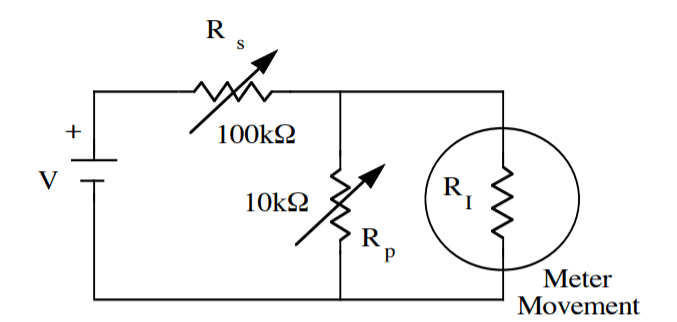# Finding Internal Resistance through Circuit Analysis

• Engineering
Safder Aree

## Homework Statement

Given this following circuit:What is the internal resistance of the meter movement ( R_I).

This is part of a project I'm doing and I know the equation that gives you the internal resistance in this circuit.
$$R_I = \frac{R_sR_p}{R_s - R_p}$$.

However, I have no idea how this is derived. I know the values for V, R_s and R_p as it was done experimentally.

## Homework Equations

$$R_I = \frac{R_sR_p}{R_s - R_p}$$.

$$V = IR$$

## The Attempt at a Solution

Using circuit analysis I can simplify the parallel portion as:
$$R_{parallel} = \frac{1}{R_p} + \frac{1}{R_I}$$

Thus:
$$R_{total} = R_s + \frac{1}{R_p} + \frac{1}{R_I}$$

But what is R_total, how do I get rid of it?

Thank you for any guidance.

Last edited:

## Answers and Replies

Mentor
Using circuit analysis I can simplify the parallel portion as:
$$R_{parallel} = \frac{1}{R_p} + \frac{1}{R_s}$$
Why do you consider those two resistances to be in parallel? Are you trying to find a Thevenin equivalent?
Thus:
$$R_{total} = R_s + \frac{1}{R_p} + \frac{1}{R_s}$$
That can't be right, the units of the terms would not match.
But what is R_total, how do I get rid of it?
Perhaps you can explain what it is you're trying to accomplish with the steps you've taken? What's your plan of attack on this problem?

Is there more information about the lab that you can share? How did you arrive at values for ##R_s## and ##R_p##? What was the procedure?

Is there more information about the meter movement that we need to know, such as the full-scale current value?

Safder Aree
Why do you consider those two resistances to be in parallel? Are you trying to find a Thevenin equivalent?

That can't be right, the units of the terms would not match.

Perhaps you can explain what it is you're trying to accomplish with the steps you've taken? What's your plan of attack on this problem?

Is there more information about the lab that you can share? How did you arrive at values for ##R_s## and ##R_p##? What was the procedure?

Is there more information about the meter movement that we need to know, such as the full-scale current value?

I made a typo. R_parallel is actually
$$R_{parallel} = \frac{1}{R_p} + \frac{1}{R_I}$$

To get ##R_s## and ##R_p## I used the values from a variable resistance box. The ##R_s## value was found first in a circuit where there was no ##R_p##, the value was what gave the meter movement full scale deflection. Then I proceeded to add ##R_p## and find the resistance for when I got half scale deflection on the meter movement.

Mentor
To get $R_s$ and $R_p$ I used the values from a variable resistance box. The $R_s$ value was found first in a circuit where there was no $R_p$, the value was what gave the meter movement full scale deflection. Then I proceeded to add $R_p$ and find the resistance for when I got half scale deflection on the meter movement.
So you need to incorporate those conditions into your analysis. Assume some meter current ##I_m## is the full-scale current value for your meter, and that deflection is proportional to current. What equations can you write for the two scenarios?

Safder Aree
So you need to incorporate those conditions into your analysis. Assume some meter current ##I_m## is the full-scale current value for your meter, and that deflection is proportional to current. What equations can you write for the two scenarios?

So I know that ##I_m## must be:
$$\frac{V}{R_s + R_I}$$?

Not sure where to go from here.

Mentor
So I know that ##I_m## must be:
$$\frac{V}{R_s + R_I}$$
Okay, that's for the first case where ##R_p## was not connected.

What's the situation for the next scenario where ##R_p## is introduced?

Safder Aree
Okay, that's for the first case where ##R_p## was not connected.

What's the situation for the next scenario where ##R_p## is introduced?

Then for the case $R_p$ is introduced, it should be one half of that value right?

Mentor
Then for the case $R_p$ is introduced, it should be one half of that value right?
That's what your additional information about the problem stated, so yes. Can you analyze the circuit to find the current through the meter for this scenario?

Safder Aree
That's what your additional information about the problem stated, so yes. Can you analyze the circuit to find the current through the meter for this scenario?

Then current is,

$$I = VR_{total}$$
$$= V (R_s + \frac{1}{R_p} + \frac{1}{R_I})$$

Mentor
Then current is,

$$I = VR_{total}$$
$$= V (R_s + \frac{1}{R_p} + \frac{1}{R_I})$$

Sorry, That makes no sense. The units do not work in that expression; You cannot sum Ohms with inverse Ohms.

Use whatever circuit analysis method you prefer to determine the current though the meter. Kirchhoff's law directly or mesh analysis, or Nodal analysis, or whatever (Thevenin equivalents, Norton equivalents...). But you need to analyze the circuit appropriately.

The total current supplied by the voltage source is not the current through the meter. You need to find an expression for the current through the meter.